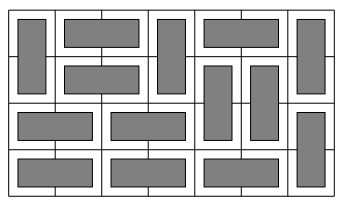# Can anyone help me with this problem?

Calculating the number of distinct ways to fill an n × m grid using 1 × 2 and 2 × 1 size tiles. For example, one valid solution for the 4 × 7 grid is and the total number of solutions is 781.This is a pretty hard problem and probably doesn’t have a DP solution. You can have a look at http://www.math.cmu.edu/~bwsulliv/domino-tilings.pdf

3 Likes

I know the solution of this problem through a mathematical formula. But, I want to know that can we solve this problem through Dynamic Programming because they have put it in Dynamic Programming section.

@admin I have also checked the resource (pdf) which you sent. But, It can be solved through Dynamic Programming.
@codechef

@admin I got one resource which can help others too!
https://stackoverflow.com/questions/31354624/number-of-ways-to-tile-a-w-x-h-grid-with-2-x-1-and-1-x-2-dominos

Thanks @admin for response1 Like

Yes, I meant no efficient (ie. poly time) DP solutions. There are numerous exponential time solutions.

1 Like

Thanks @admin